PHP Training

### Data Types and Operators

#### PHP Arrays

An array stores multiple values in one single variable:

### Example

<?php
\$cars = array("Volvo", "BMW", "Toyota");
echo "I like " . \$cars . ", " . \$cars . " and " . \$cars . ".";
?>
//Output:
I like Volvo, BMW and Toyota.

#### What is an Array?

An array is a special variable, which can hold more than one value at a time.

If you have a list of items (a list of car names, for example), storing the cars in single variables could look like this:

\$cars1 = "Volvo";
\$cars2 = "BMW";
\$cars3 = "Toyota";

However, what if you want to loop through the cars and find a specific one? And what if you had not 3 cars, but 300?

The solution is to create an array!

An array can hold many values under a single name, and you can access the values by referring to an index number.

#### Create an Array in PHP

In PHP, the array() function is used to create an array:

array();

In PHP, there are three types of arrays:

Indexed arrays - Arrays with a numeric index

Associative arrays - Arrays with named keys

Multidimensional arrays - Arrays containing one or more arrays

#### PHP Indexed Arrays

There are two ways to create indexed arrays:

The index can be assigned automatically (index always starts at 0), like this:

\$cars = array("Volvo", "BMW", "Toyota");

or the index can be assigned manually:

\$cars = "Volvo";
\$cars = "BMW";
\$cars = "Toyota";

The following example creates an indexed array named \$cars, assigns three elements to it, and then prints a text containing the array values:

### Example

<?php
\$cars = array("Volvo", "BMW", "Toyota");
echo "I like " . \$cars . ", " . \$cars . " and " . \$cars . ".";
?>
//Output:
I like Volvo, BMW and Toyota.

#### Get The Length of an Array - The count() Function

The count() function is used to return the length (the number of elements) of an array:

### Example

<?php
\$cars = array("Volvo", "BMW", "Toyota");
echo count(\$cars);
?>

#### Loop Through an Indexed Array

To loop through and print all the values of an indexed array, you could use a for loop, like this:

### Example

<?php
\$cars = array("Volvo", "BMW", "Toyota");
\$arrlength = count(\$cars);

for(\$x = 0; \$x < \$arrlength; \$x++) {
echo \$cars[\$x];
echo "<br>";
}
?>

#### PHP Associative Arrays

Associative arrays are arrays that use named keys that you assign to them.

There are two ways to create an associative array:

\$age = array("Peter"=>"35", "Ben"=>"37", "Joe"=>"43");

or:

\$age['Peter'] = "35";
\$age['Ben'] = "37";
\$age['Joe'] = "43";

The named keys can then be used in a script:

### Example

<?php
\$age = array("Peter"=>"35", "Ben"=>"37", "Joe"=>"43");
echo "Peter is " . \$age['Peter'] . " years old.";
?>

#### Making fetch Parameter Using Associative array

```    \$actors = \$blActor->GetMany(array('order_by'=>'actor_id','columns'=>'fname.lname,id', 'where'=>array('fname'=>\$fname, lname=>\$lname));
OR

\$conditionArray=array('order_by'=>\$_GET["orderby"], 'columns'=> ' first_name, last_name, actor_id ');
if(\$fname!="" || \$lname!="" )
{
if(\$fname!="")
\$condition['lower(first_name)']=strtolower(\$fname);
if(\$lname!="")
\$condition['lower(last_name)']=strtolower(\$lname);
//array_push(\$conditionArray, 'where'=>array('lower(first_name)'=>strtolower(\$filterFName)));
OR
\$conditionArray['where']=\$condition;
}

\$actors = \$blActor->GetMany(\$conditionArray);
```

#### Loop Through an Associative Array

To loop through and print all the values of an associative array, you could use a foreach loop, like this:

### Example

<?php
\$age = array("Peter"=>"35", "Ben"=>"37", "Joe"=>"43");

foreach(\$age as \$x => \$x_value) {
echo "Key=" . \$x . ", Value=" . \$x_value;
echo "<br>";
}
?>

#### PHPSorting Arrays

The elements in an array can be sorted in alphabetical or numerical order, descending or ascending.

#### PHP - Sort Functions For Arrays

In this chapter, we will go through the following PHP array sort functions:

sort() - sort arrays in ascending order

rsort() - sort arrays in descending order

asort() - sort associative arrays in ascending order, according to the value

ksort() - sort associative arrays in ascending order, according to the key

arsort() - sort associative arrays in descending order, according to the value

krsort() - sort associative arrays in descending order, according to the key

#### Sort Array in Ascending Order - sort()

The following example sorts the elements of the \$cars array in ascending alphabetical order:

### Example

<?php
\$cars = array("Volvo", "BMW", "Toyota");
sort(\$cars);
?>

The following example sorts the elements of the \$numbers array in ascending numerical order:

### Example

<?php
\$numbers = array(4, 6, 2, 22, 11);
sort(\$numbers);
?>

#### Sort Array in Descending Order - rsort()

The following example sorts the elements of the \$cars array in descending alphabetical order:

### Example

<?php
\$cars = array("Volvo", "BMW", "Toyota");
rsort(\$cars);
?>

The following example sorts the elements of the \$numbers array in descending numerical order:

### Example

<?php
\$numbers = array(4, 6, 2, 22, 11);
rsort(\$numbers);
?>

#### Sort Array (Ascending Order), According to Value - asort()

The following example sorts an associative array in ascending order, according to the value:

### Example

<?php
\$age = array("Peter"=>"35", "Ben"=>"37", "Joe"=>"43");
asort(\$age);
?>

#### Sort Array (Ascending Order), According to Key - ksort()

The following example sorts an associative array in ascending order, according to the key:

### Example

<?php
\$age = array("Peter"=>"35", "Ben"=>"37", "Joe"=>"43");
ksort(\$age);
?>

#### Sort Array (Descending Order), According to Value - arsort()

The following example sorts an associative array in descending order, according to the value:

### Example

<?php
\$age = array("Peter"=>"35", "Ben"=>"37", "Joe"=>"43");
arsort(\$age);
?>

#### Sort Array (Descending Order), According to Key - krsort()

The following example sorts an associative array in descending order, according to the key:

### Example

<?php
\$age = array("Peter"=>"35", "Ben"=>"37", "Joe"=>"43");
krsort(\$age);
?>

Check Example Here: Adding and getting objects to and from Array

```
class Products
{
/* Member variables */
var \$name;
var \$price;
var \$color;

function __construct( \$par1, \$par2,\$color ) {
\$this->name = \$par1;
\$this->price = \$par2;
\$this->color = \$color;
}
}
\$product1=new Products('Kurti chikan',100,'Grey');
\$productList = array(\$product1,new Products('Kurti',100, 'orange'), new Products('Salwar Suit',200,'Black'));
array_push(\$productList, new Products('Dupatta',300,'Red'));
array_push(\$productList, new Products('t-Shirt',500,'Green'));
```

#### PHP 5 Array Functions

Function Description
array() Creates an array
array_change_key_case() Changes all keys in an array to lowercase or uppercase
array_chunk() Splits an array into chunks of arrays
array_column() Returns the values from a single column in the input array
array_combine() Creates an array by using the elements from one "keys" array and one "values" array
array_count_values() Counts all the values of an array
array_diff() Compare arrays, and returns the differences (compare values only)
array_diff_assoc() Compare arrays, and returns the differences (compare keys and values)
array_diff_key() Compare arrays, and returns the differences (compare keys only)
array_diff_uassoc() Compare arrays, and returns the differences (compare keys and values, using a user-defined key comparison function)
array_diff_ukey() Compare arrays, and returns the differences (compare keys only, using a user-defined key comparison function)
array_fill() Fills an array with values
array_fill_keys() Fills an array with values, specifying keys
array_filter() Filters the values of an array using a callback function
array_flip() Flips/Exchanges all keys with their associated values in an array
array_intersect() Compare arrays, and returns the matches (compare values only)
array_intersect_assoc() Compare arrays and returns the matches (compare keys and values)
array_intersect_key() Compare arrays, and returns the matches (compare keys only)
array_intersect_uassoc() Compare arrays, and returns the matches (compare keys and values, using a user-defined key comparison function)
array_intersect_ukey() Compare arrays, and returns the matches (compare keys only, using a user-defined key comparison function)
array_key_exists() Checks if the specified key exists in the array
array_keys() Returns all the keys of an array
array_map() Sends each value of an array to a user-made function, which returns new values
array_merge() Merges one or more arrays into one array
array_merge_recursive() Merges one or more arrays into one array recursively
array_multisort() Sorts multiple or multi-dimensional arrays
array_pad() Inserts a specified number of items, with a specified value, to an array
array_pop() Deletes the last element of an array
array_product() Calculates the product of the values in an array
array_push() Inserts one or more elements to the end of an array
array_rand() Returns one or more random keys from an array
array_reduce() Returns an array as a string, using a user-defined function
array_replace() Replaces the values of the first array with the values from following arrays
array_replace_recursive() Replaces the values of the first array with the values from following arrays recursively
array_reverse() Returns an array in the reverse order
array_search() Searches an array for a given value and returns the key
array_shift() Removes the first element from an array, and returns the value of the removed element
array_slice() Returns selected parts of an array
array_splice() Removes and replaces specified elements of an array
array_sum() Returns the sum of the values in an array
array_udiff() Compare arrays, and returns the differences (compare values only, using a user-defined key comparison function)
array_udiff_assoc() Compare arrays, and returns the differences (compare keys and values, using a built-in function to compare the keys and a user-defined function to compare the values)
array_udiff_uassoc() Compare arrays, and returns the differences (compare keys and values, using two user-defined key comparison functions)
array_uintersect() Compare arrays, and returns the matches (compare values only, using a user-defined key comparison function)
array_uintersect_assoc() Compare arrays, and returns the matches (compare keys and values, using a built-in function to compare the keys and a user-defined function to compare the values)
array_uintersect_uassoc() Compare arrays, and returns the matches (compare keys and values, using two user-defined key comparison functions)
array_unique() Removes duplicate values from an array
array_unshift() Adds one or more elements to the beginning of an array
array_values() Returns all the values of an array
array_walk() Applies a user function to every member of an array
array_walk_recursive() Applies a user function recursively to every member of an array
arsort() Sorts an associative array in descending order, according to the value
asort() Sorts an associative array in ascending order, according to the value
compact() Create array containing variables and their values
count() Returns the number of elements in an array
current() Returns the current element in an array
each() Returns the current key and value pair from an array
end() Sets the internal pointer of an array to its last element
extract() Imports variables into the current symbol table from an array
in_array() Checks if a specified value exists in an array
key() Fetches a key from an array
krsort() Sorts an associative array in descending order, according to the key
ksort() Sorts an associative array in ascending order, according to the key
list() Assigns variables as if they were an array
natcasesort() Sorts an array using a case insensitive "natural order" algorithm
natsort() Sorts an array using a "natural order" algorithm
next() Advance the internal array pointer of an array
pos() Alias of current()
prev() Rewinds the internal array pointer
range() Creates an array containing a range of elements
reset() Sets the internal pointer of an array to its first element
rsort() Sorts an indexed array in descending order
shuffle() Shuffles an array
sizeof() Alias of count()
sort() Sorts an indexed array in ascending order
uasort() Sorts an array by values using a user-defined comparison function
uksort() Sorts an array by keys using a user-defined comparison function
usort() Sorts an array using a user-defined comparison function

### PHP Workflow

( 0 Review )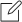### Course Code :

02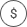### Price :

5000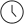### Duration :

30 days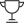### Lectures :

10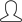### Students :

10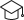### Certificate of Completion### Manish Kumar Shrivastava

Web Developer

My name is Ruth. I grew up and studied in…